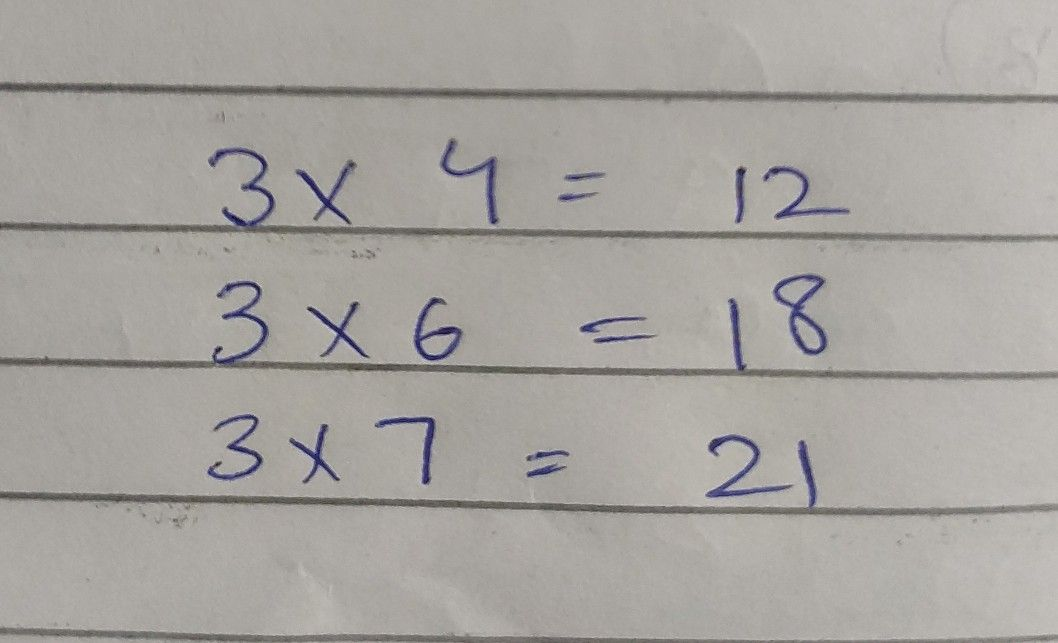Symbol
Problem$3$ times $4$ is $12$ $-$ $3\times 4=$ $12$ $3$ times $6$ is $10$ $3\times 6=$ $18$ 0 00 $3$ times $7$ is $2|$ $3\times 7=$ $21$